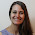## Elementary Studies

### Divisibility Rules

Divisible: When one number can be divided by another number without leaving a remainder.
For example, 6 is divisible by 3.

Divisibility rules of whole numbers help us to quickly determine if a number can be divided by 2, 3, 4, 5, 9, and 10 without doing a long division. These rules have a wide range of applications in mathematics like finding factors, determining prime versus composite numbers and simplifying fractions etc.

Knowing the divisibility rules will prepare students to work with larger numbers. You may introduce the concept in grade 4 and as a refresher for 5th graders.

Use these worksheets and a ready reference poster to have students familiarize and practice the divisibility rules. Included are 11 worksheets and 2 posters (both in Colored and Black & White versions) for dividing by 2, 3, 4, 5, 6, 9, and 10.
You may also print the poster as 2 copies per page and have students stick in their notebooks for quick reference.

You can buy this Divisibility Rules pack from HERE.

Thanks

Shimps

#### 1 comment:

1.This looks like a great resource for teaching divisibility!